Identifying Congruent Parts
Congruence Statements
Name that Theorem
Challenge
Identify the Theorem
100

Given the following statement, which angle is congruent to angle W?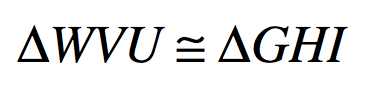Angle G

100

Finish the congruence statement for the two triangles given: triangle ABC is Congruent to triangle ______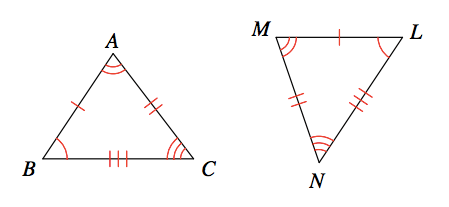triangle MLN

100

Two triangles where we know all three sides are congruent.

Side-Side-Side (SSS)

100

What does CPCTC stand for?

Corresponding parts of congruent triangles are congruent

100

Which rule proves these triangles are congruent?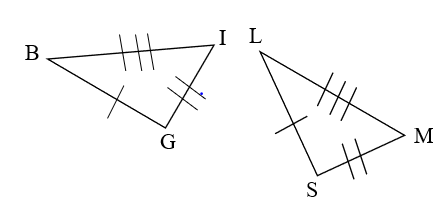SSS

200

Given the following statement, which side is congruent to VU?HI

200

Finish the congruence statement for the two triangles given: triangle EDF is Congruent to triangle ______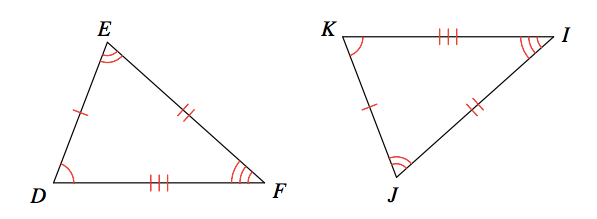triangle JKI

200

Two triangles where we know two angles and the included side are congruent

Angle-Side-Angle (ASA)

200

Given that the triangles are congruent, find the value of x.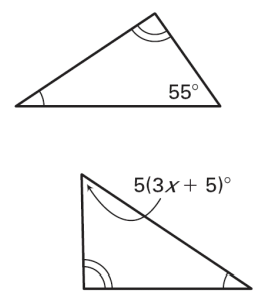x=2

200

Which rule proves these triangles are congruent?SAS

300

Given the following statement, which side is congruent to IG?UW

300

Finish the congruence statement for the two triangles given: triangle BCD is Congruent to triangle ______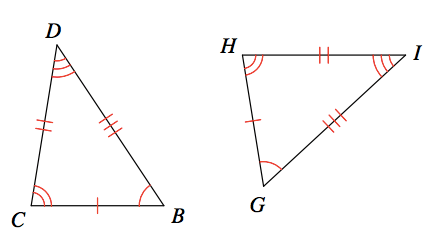triangle GHI

300

Two triangles where we know two sides and the included angle are congruent.

Side-Angle-Side (SAS)

300

Which rule proves these triangles are congruent?AAS

300

Which rule proves these triangles are congruent?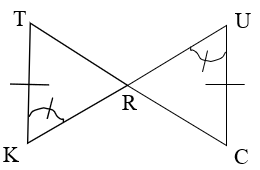AAS

400

Use the diagram to identify which side is congruent to CB.NL

400

Finish the congruence statement for the two triangles given: triangle QRP is Congruent to triangle ______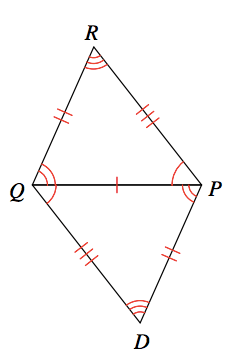triangle PDQ

400

Two triangles where we know the hypotenuse and one leg are congruent

Hypotenuse-Leg (HL)

400

Which rule proves these triangles are congruent?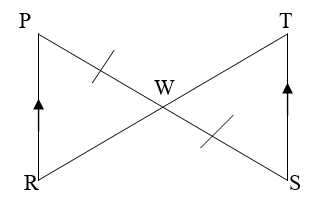AAS

400

Which rule proves these triangles are congruent?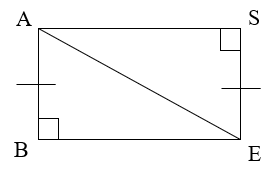HL

500

Use the diagram to determine which side is congruent to ML.AB

500

Finish the congruence statement for the two triangles given: triangle IRS is Congruent to triangle ______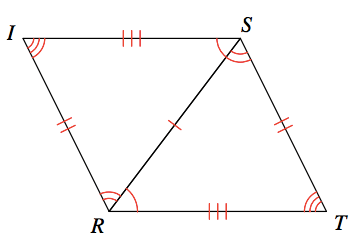triangle TSR

500

Two triangles where we know two angles and the non-included side are congruent.

Angle-Angle-Side (AAS)

500

Which rule proves these triangles are congruent?SSS

500

Which rule proves these triangles are congruent?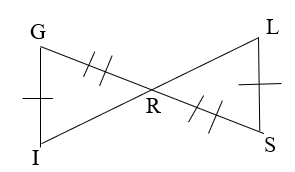None

Click to zoom